Computer Science

# Variables and Functions

Today we are reviewing some of the basics to be sure everyone understands what we have been doing so far.

## while loops

``````from byubit import Bit

bit = Bit.new_world(5, 3)
bit.draw()``````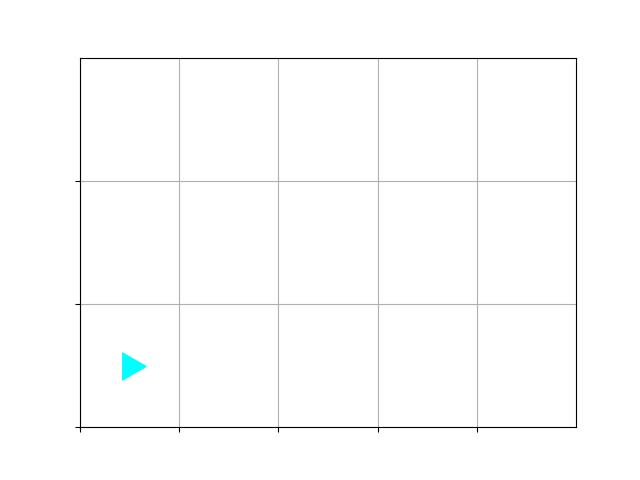### Post-condition for a while loop

If we want to paint the bottom row green, we can use the following:

``````while bit.front_clear():
bit.paint("green")
bit.move()
bit.draw()``````

However, notice that we are missing the last block: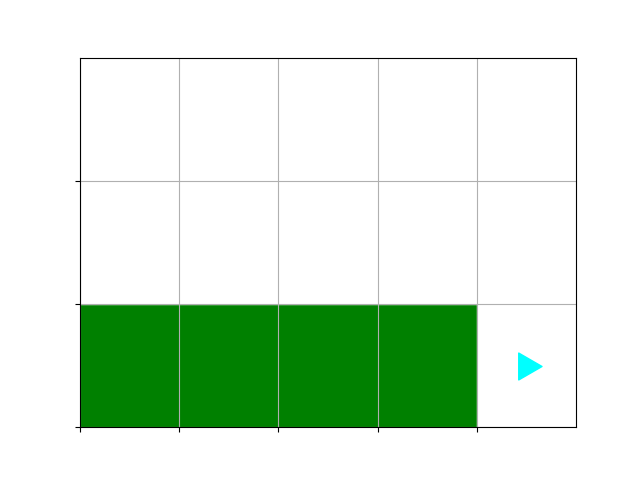This is a great example of a post-condition. After the while loop ends, bit is sitting on the last square, and it is not painted. How do we paint the last square? Add an extra paint() after the while loop:

``````while bit.front_clear():
bit.paint("green")
bit.move()
bit.paint("green")
bit.draw()``````

### Pre-condition for a while loop

Likewise, if we swap the paint() and move() functions, we get this:

``````while bit.front_clear():
bit.move()
bit.paint("green")
bit.draw()``````

Now, the first square is not painted. Think about the pre-condition for the while loop. Bit starts on an unpainted square. The first thing we do in the while loop is move to the next square. So to paint the first square, add an extra paint() before the while loop:

``````bit.paint("green")
while bit.front_clear():
bit.move()
bit.paint("green")
bit.draw()``````

## Variables

Let’s create a way to paint whatever color we want:

``````color = "blue"
while bit.front_clear():
bit.paint(color)
bit.move()
bit.paint(color)``````

Play with this code in PyCharm. Notice how you can change the value of the variable called `color` to be “green”, “red”, or “blue”, and it paints a different color each time.

A variable has a name, a value, and a type. The name of this variable is “color”. The value of the variable is “green” (or whatever other color you choose). The type of the variable is “string” because it holds a string.

Let’s look at some other variable types:

``````name = "Sarah"
age = 23
print(name)
print(age)
``````Sarah
23
15.5``````

Here, the variable called “name” stores a string, the variable called “age” stores an integer, and the variable called “credits” stores a floating point number.

Let’s see what happens when we add 1 to each variable:

``print(name + 1)``

We get a type error:

``````Traceback (most recent call last):
File "/Users/zappala/Documents/CS110/class/paint.py", line 19, in <module>
print(name + 1)
TypeError: can only concatenate str (not "int") to str``````

We can’t use the ”+” operator to add a string and an integer. But the others will work:

``````print(age + 1)
``````24
16.1``````

We can use the ”+” operator to add two or more strings:

``````first = "Sarah"
last = "Jones"
print(first + " " + last)``````

### Multiplying

Let’s try multiplying:

``````print(name*2)
print(age*2)
``````SarahSarah
46
31.0``````

So we can also multiply strings. In fact, we can multiply them many times:

``print(name*100)``

Sometimes we will call this “duck typing”. This term comes from the saying If it walks like a duck, and it quacks like a duck, then it must be a duck.

Basically, if an operation (such as multiplication) is defined for a given type, then it can be used for that type (such as multiplying strings).

## Functions

Functions allow us to give a name to a set of statements. For example:

``````def happy():
print("I'm feeling happy")

happy()
happy()
happy()``````

This defines a function called `happy()`, which just prints a simple statement.Running this will get us:

``````I'm feeling happy
I'm feeling happy
I'm feeling happy``````

### Parameters (or arguments)

We can also pass parameters to a function:

``````def say_how_i_am_feeling(feeling):
# I'm going to print how I'm feeling
print("I'm feeling")
# I get the feeling from a variable called feeling
print(feeling)

my_feeling = "quite impressed"
say_how_i_am_feeling(my_feeling)``````

In this example, the variable `my_feeling` is set to a string, and then passed as a parameter to the `say_how_i_am_feeling()` function. Inside this function, the variable is called simply `feeling`.

We could do this in one step with:

``say_how_i_am_feeling("quite impressed")``

In this case, the string “quite impressed” is stored in the variable `feeling` once it is passed to the function.

In both cases, this will print:

``````I'm feeling
quite impressed``````

### String interpolation

Remember that we can use string interpolation to print all of this on one line, like this:

``````def say_how_i_am_feeling(feeling):
# I'm going to print how I'm feeling
print(f"I'm feeling {feeling}")

my_feeling = "quite impressed"
say_how_i_am_feeling(my_feeling)``````

Notice that we use a single character, `f` at the start of the string, and then we can put variables inside curly brackets, like `{feeling}`.

### Docstrings, pre-conditions and post-conditions

When you write functions, you should always include a docstring, which is surrounded by `"""`. You can include the pre-conditions and post-conditions for the function in this docstring. For example:

``````def say_how_i_am_feeling(feeling):
"""
This is a function that prints how someone is feeling.

Pre-condition: The variable feeling stores a string
Post-condition: The console shows a printed statement that says how I am feeling

"""
# I'm going to print how I am feeling
print(f"I'm feeling {feeling}")``````

## Bit and functions

Let’s take a look at the tree problem again. Put the following into a file called `tree.txt`:

``````-------------
-ggggggggggg-
---ggggggg---
-------------
-------------
-------------
-------------
-------------
------r------
kkkkkkkkkkkkk
0 1
0``````

The top part sets up the world for Bit (green is “g”, red is “r”, black is “k”), and the bottom part shows the position of Bit and its direction.

``````from byubit import Bit
bit.draw()``````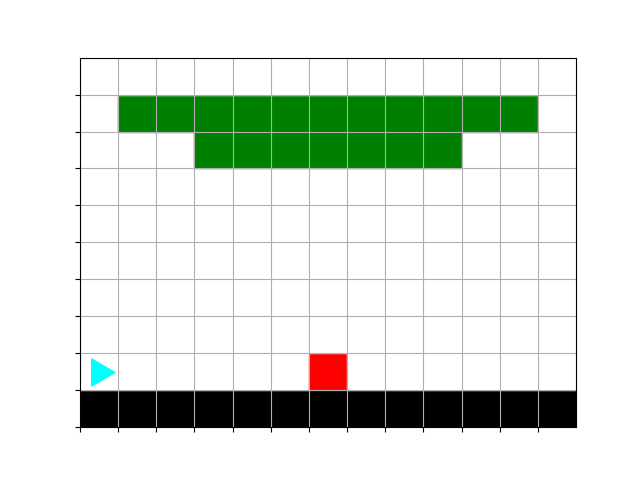### Variables that store a bit world

Let’s pause right here for a moment. What is `bit` in the code above? It’s a variable! And it stores a bit world. In fact, it can be any name we want:

``````from byubit import Bit
world.draw()``````

### Moving to the trunk

Let’s write a function that will move Bit until it reaches the bottom of the trunk:

``````from byubit import Bit

def move_to_trunk(bit):
""" Moves to the trunk, stopping at the first red square """
while bit.get_color() != "red":
bit.move()

move_to_trunk(world)
world.draw()``````

OK, this puts together a few things we have learned:

• `world` is a variable that holds the Bit world.
• When we call `move_to_trunk()`, we give it `world`, and inside the function this is stored in a variable called `bit`.
• We can use a while loop to move to the bottom of the tree.

This gets us here: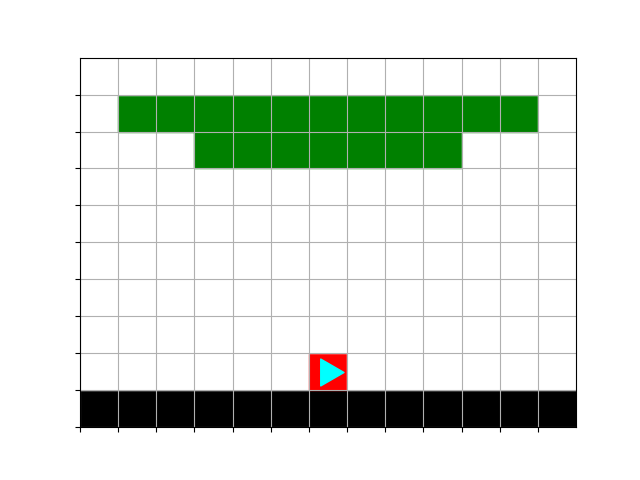### Revisiting variables

Remember that `bit` and `world` are just variables that hold a Bit world (instead of a string or an integer). Put the following in a file called `mountain.txt`:

``````--------r----
-------rrr---
------rrrrr--
kkkkkkkkkkkkk
0 1
0``````

This world looks like this: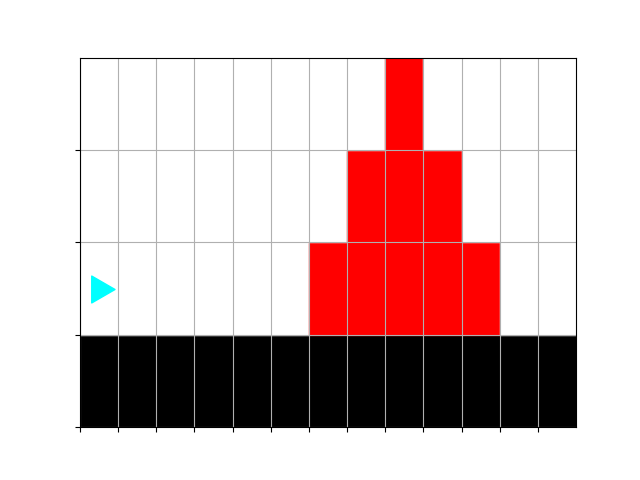Now let’s try this:

``````from byubit import Bit

def move_to_trunk(bit):
""" Moves to the trunk, stopping at the first red square """
while bit.get_color() != "red":
bit.move()

move_to_trunk(world1)
world1.draw()

move_to_trunk(world2)
world2.draw()``````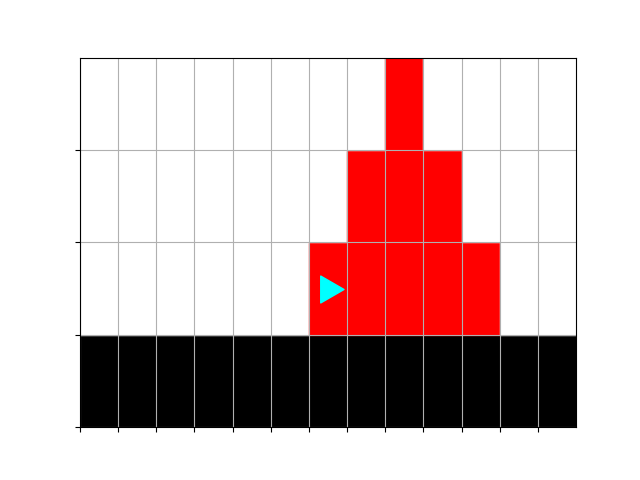The same `move_to_trunk()` function works fine! This is because all it needs is a bit world, and it doesn’t care if it is given the `world1` variable or the `world2` variable.

### Decomposition

Let’s finish with a little bit of decomposition. We already have a function that can move to the trunk. Let’s write another function that can draw the trunk:

``````def draw_trunk(bit):
""" Draws a trunk, stopping at the first green square. """
bit.left()
while bit.get_color() != "green":
bit.paint("red")
bit.move()``````

We can combine these two:

``````from byubit import Bit

def move_to_trunk(bit):
""" Moves to the trunk, stopping at the first red square """
while bit.get_color() != "red":
bit.move()

def draw_trunk(bit):
bit.left()
while bit.get_color() != "green":
bit.paint("red")
bit.move()

move_to_trunk(world)
draw_trunk(world)
world.draw()``````

This gets us: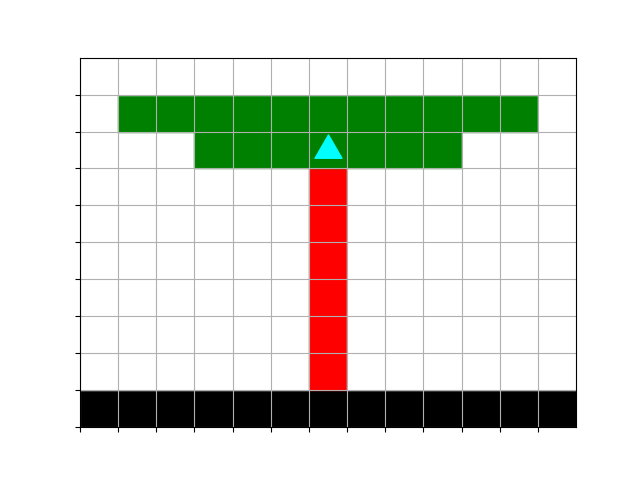### Multiple functions

And we can call functions from other functions! For example:

``````from byubit import Bit

def move_to_trunk(bit):
""" Moves to the trunk, stopping at the first red square """
while bit.get_color() != "red":
bit.move()

def draw_trunk(bit):
bit.left()
while bit.get_color() != "green":
bit.paint("red")
bit.move()

def fix_tree(bit):
move_to_trunk(bit)
bit.draw()
draw_trunk(bit)
bit.draw()

fix_tree(world)``````

## More practice

Keep working on decomposition. For example, you might try the `fix-the-forest` problem, using this file in `forest.txt`:

``````------------ggggggg------------
--ggggg------ggggg-------------
---ggg----g-----------ggggg----
---------ggg-------ggggggggggg-
----------g------------ggg-----
-------------------------------
-------------------------------
----r-----r----r--------r------
kkkkkkkkkkkkkkkkkkkkkkkkkkkkkkk
0 1
0``````

You need:

• a function that goes back down the tree and stops just to the right of the trunk
• a function to fix all the trees